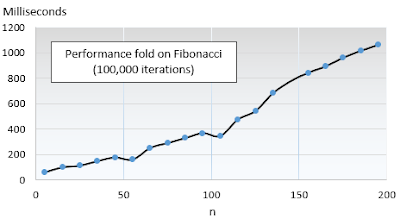## Thursday, July 9, 2015

### Fibonacci Recursive Implementation Galore

This post evaluates the performance of the tail recursion in Scala relative to alternative solutions as applied to the Fibonacci formula

Overview
There are many ways to skin a cat and implement the Fibonacci recursion. This post illustrates the power of tail recursion in Scala, relative to alternative solution such as recursion without tail elimination and iterations. This post compares the performance of the simple "out-of-the-box" implementation of the Fibonacci recursion with an Scala implementation using tail-recursion.

Performance of non-tail recursive Fibonacci
The Fibonacci algorithm is defined as
   f(n) = f(n-1) + f(n-2)
f(0) = 0
f(1) = 1

Let's look at text book recursive implementation.

def fibonacci(n: Int): Long =  {
if(n == 0) 0
else if(n == 1) 1
else fibonacci1(n-1) + fibonacci1(n-2)
}


The duration of execution is recorded for values between 2 and 50, run 10,000 times.The profile of the performance of the non-tail recursion of Fibonacci should not be a surprise. After all, the time complexity of this implementation is a staggering O(2n)

Tail-recursive implementation
Let's look at one example of implementation of the Fibonacci formula using the Scala tail recursion.

def fibonacci(n: Int): Long = {
@tailrec
def _fibonacci(i: Int, next: Long, sum: Long): Long = {
if(i ==0) sum
else fibonacci(i-1, sum+ next, next)
}
fibonacci(n, 1, 0)
}


The performance of the tail recursion is measure against a plain-vanilla iterative execution. The duration of execution is recorded for values between 5 and 200, run 100,000 times.

def fibonacci(n: Int): Long = {
var s1 = 0
var s2 = 1
(n-1 to 0 by -1).foreach(_ => {
val tmp = s2 + s1
s1 = s2
s2 = tmp
})
s1
}

br>
The following chart illustrates that the performance of the implementation of Fibonacci using the tail recursion and the iterative execution is almost identical.Implementation of iterative computation
For good measure, I thought it would be interesting to measure the performance of an iterative approach using a fold.

def fibonacci(n: Int): Long =
(n-1 to 0 by -1)./:((0, 1)){ case ((s1, s2), n) => {
val tmp = s2 + s1
(s2, tmp)
}}._1


Here is the performance profile for the iterative computation.Note: The fold (:/) can be implemented as a closure using a recursive formula for the function argument.

Wrapping up
The Scala tail recursion is indeed as effective as the iterative implementation at processing the Fibonacci formula. The performance of the recursion without tail elimination is extremely poor. Aggregators or reducers such as fold, reduce and scan add some overhead to the computation although the performance is still decent.

Reference